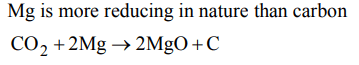## The s-Block Elements Questions and Answers Part-17

1. Hesenclever’s process is a method for the manufacture of
a) NaOH
b) $HNO_{3}$
c) $H_{2}SO_{4}$
d) Bleaching powder

Explanation: Hasenclever and Batchmann’s plants are used for the manufacture of bleaching powder

2. Oxygen is obtained from bleaching powder by
a) the action of dilute acid
b) the action of alkali
c) heating it with lime
d) heating it with cobalt salt

Explanation:3. Portland cement does not contain
a) $CaSiO_{4}$
b) $Ca_{2}SiO_{5}$
c) $Ca_{2}Al_{2}O_{6}$
d) $Ca_{3}\left(PO_{4}\right)_{2}$

Explanation: Portland cement is a complex mixture of silicates and aluminates of Ca

4. Alkaline earth metal compounds are less soluble in water than corresponding alkali metal compounds because former have
a) lower lattice energy
b) higher I.P.
c) higher covalent character
d) lower covalent character

Explanation: The higher the covalent character, the lower the solubility of compound in water

5. Magnesium wire burns in the atmosphere of $CO_{2}$ because
a) Magnesium acts as an oxidising agent
b) Magnesium has 2 electrons in the outermost orbit
c) Magnesium acts as a reducing agent and removes oxygen from $CO_{2}$
d) None of these

Explanation:6. When hydrated $MgCl_{2}.6H_{2}O$    is strongly heated
a) MgO is formed
b) Mg$\left(OH\right)_{2}$  is formed
c) Mg(OH)Cl is formed
d) Anhydrous $MgCl_{2}$  is formed

Explanation:7. Which component of cement sets at the slowest rate
a) Dicalcium silicate
b) Tricalcium silicate
c) Tricalcium aluminate
d) Tetracalcium aluminoferrite

Explanation: Dicalcium silicate sets at the lowest rate

8. The cation which forms a yellow precipitate with potassium chromate in acetic acid is
a) $NH_4^+$
b) $Ba^{2+}$
c) $Ca^{2+}$
d) $Na^{+}$

Explanation: Ba++ with CrO4-- give yellow BaCrO4 . It is insoluble in CH3COOH

9. The ionic carbide is
a) $CaC_{2}$
b) CaO
c) $Ca\left(OH\right)_{2}$
d) $Ca\left(HCO_{3}\right)_{2}$